Wang, Z., Wang, Z., Wang, B. J., Wang, Y., Liu, B., Rao, X., Wei, P., and Yang. Y. (2014). "Dynamic testing and evaluation of modulus of elasticity (MOE) of SPF dimensional lumber

#### Abstract

The key objective of this work was to develop a cost-effective and reliable non-destructive testing (NDT) method to measure lumber modulus of elasticity (MOE). Test samples comprised 300 pieces of randomly selected imported Canadian 2”×6” SPF (Spruce – lodgepole Pine – alpine Fir) dimensional lumber. This work first adopted a modal test to achieve a free suspension for a subsequent vibration test. Then, the first-order natural frequency of 300 SPF dimensional lumber specimens was measured by the transient excitation frequency method, based on which MOE was then calculated. The results were also validated by a stress wave method using a Hitman tool. The measured lumber MOE data were further fitted using a Weibull distribution. The results showed that the MOE of SPF dimensional lumber followed either a three-parameter Weibull distribution or a normal distribution. It was estimated that the probability that the MOE of this SPF dimensional lumber was less than 8,000 MPa was about 13.8% and 13.6%, respectively, using the above two distributions.

Dynamic Testing and Evaluation of Modulus of Elasticity (MOE) of SPF Dimensional Lumber

Zhiheng Wang,a Zheng Wang,a,* Brad J. Wang,b Yalei Wang,c Bin Liu,c Xin Rao,d Peixing Wei,a and Yan Yang c

The key objective of this work was to develop a cost-effective and reliable non-destructive testing (NDT) method to measure lumber modulus of elasticity (MOE). Test samples comprised 300 pieces of randomly selected imported Canadian 2”×6” SPF (Spruce – lodgepole Pine – alpine Fir) dimensional lumber. This work first adopted a modal test to achieve a free suspension for a subsequent vibration test. Then, the first-order natural frequency of 300 SPF dimensional lumber specimens was measured by the transient excitation frequency method, based on which MOE was then calculated. The results were also validated by a stress wave method using a Hitman tool. The measured lumber MOE data were further fitted using a Weibull distribution. The results showed that the MOE of SPF dimensional lumber followed either a three-parameter Weibull distribution or a normal distribution. It was estimated that the probability that the MOE of this SPF dimensional lumber was less than 8,000 MPa was about 13.8% and 13.6%, respectively, using the above two distributions.

Key words: Dimensional lumber; Modulus of elasticity (MOE); Natural frequency; Stress wave; Transverse vibration; Weibull distribution; Normal distribution

Contact information: a: College of Wood Science and Technology, Nanjing Forestry University, Nanjing, 210037, China; b: FPInnovations-Wood Products, Vancouver, BC V6T1W5 Canada; c: College of Mechatronics Engineering, Nanjing Forestry University, Nanjing, 210037, China; d: Jiangsu Polytechnic College Of Agriculture and Forestry, Zhenjiang, 212400, China;

* Corresponding author: wangzheng63258@163.com

INTRODUCTION

Non-destructive testing (NDT) technology has been widely used in measuring various materials, such as iron, steel, alloy, non-steel metal, polymer, ceramics, glass, cement, concrete, lumber, and composites (Zheng et al. 2002). A significant effort has been devoted toward the discovery and development of NDT/NDE technologies for use with wood-based products (Brashaw et al. 2009). The application of this NDT technology in lumber dates back to the 1950s. For lumber, the modulus of elasticity (MOE) is an important parameter for its structural applications. In the testing, MOE is measured with ultrasonic or stress wave and transverse vibration technologies. Ross et al. (1999) studied the MOE of logs and lumber sawn from them, as well as the quality of rotary-cut veneer using NDT technology. The result showed that the log MOE and stress wave propagation time have a significant correlation with the MOE of the sawn lumber and stress wave propagation time of the veneer, respectively. Research on the quality of 84 commercial timbers of radiata pine showed that MOE acoustic technology was satisfactorily effective in the selection of structural logs with a diameter above two inches (Ridoutt et al. 1999). Wagner et al. (2000) conducted an analytical investigation on logs and veneers of Douglas-fir, which showed that the stress wave technology can effectively measure the MOE of structural lumber and veneer for quality assurance. In 2001, Green et al. measured the MOE of Douglas-fir structural lumber recycled from military industrial construction and found a good correlation between the dynamic MOE detected with stress wave and the static MOE and static bending strength of lumber. Therefore, the automatic classification of lumber based on MOE and static bending strength can be realized. Wang et al. (2007) took advantage of the static bending method, transverse vibration method, and longitudinal stress wave method to measure the MOE of small diameter jack pine (n=109) and red pine (50) logs, and found that the correlation between these methods can be quite high. That same year, Wang (2007) adopted a free end cantilever to measure dynamic MOE and damping ratio of high density fiberboard (HDF) and oriented strand board (OSB) samples. The results showed that the dynamic MOE is well correlated with the static MOE, and the dynamic test is fast and convenient with good repeatability. A NDT of lumber MOE can help sort lumber in real time. Besides, Yin et al. (2007) investigated the possibility of a transverse vibration technique for assessing static bending, tensile and compressive strength of structural lumbers. It was demonstrated that the transverse vibration technique can be used to predict bending and compressive properties of structural lumber. Thus, years of practice has shown that NDT is effective for MOE and strength measurement of lumber (Brown et al. 1997; Zhu et al. 2010).

Spruce, lodgepole pine, and alpine fir (SPF) dimensional lumber is widely used in light-frame construction in North America and is increasingly used in China for building applications. The MOE of SPF lumber is an important criterion for determining specific structural applications. Therefore, it is very important to find an easy, cost-effective NDT method to measure lumber MOE and analyze MOE distribution for assigning design values. The key objective of this study was to develop a cost-effective and reliable NDT method to measure the MOE of lumber. In this work, 300 representative pieces of 2×6 SPF dimensional lumber were randomly selected. A free beam vibration method was adopted to measure the lumber MOE; previously, a modal test was used to achieve free beam excitation. The test results were also validated using a stress wave method using a Hitman tool. The data was further fit to a Weibull (Weibull 1951) distribution and a normal distribution. Finally, the reliability of the MOE of the SPF dimensional lumber was estimated for a given range from 8,000 MPa to 9,000 MPa.

EXPERIMENTAL

Materials

Samples tested were selected from SPF dimensional lumber pieces. The specimens from Canada used in this study possessed a good surface, close grain with small knots, and dimensional stability. A total of 300 pieces of imported Canadian 2’x6’ SPF dimensional lumber were randomly selected from storage at Nanjing Cogent Home Manufactory Ltd. The lumber was graded as Level IIc and had the dimensions of 3670 mm (L)×138 mm (b)×37 mm (h) with an air-dry density of ρ=390 to 492 kg/m3and a moisture content (MC) of 11 to 13%. Method for testing moisture content of wood is according to “GB 1931-1991 Method for determination of the moisture content of wood”.

Methods

To measure the actual air-dry density of this batch of SPF lumber, every piece was weighed, and the length, width, and height were measured, to calculate its density according to GB/T1933 (2009). The lumber MOE was measured using an instantaneous aroused frequency method. In general, the natural frequency of the first bending mode of a free beam can be calculated according to the transverse bending theory (Cheng and Timoshenko 1965):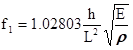(1)

With a given size of test lumber and its known density, MOE can be calculated with natural frequency f1 of the first bending mode of a free beam,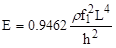(Pa) (2)

where E is the dynamic MOE of the test lumber (Pa), ρ is the air-dry density of the test lumber (kg/m3), f1 is the base frequency of the test lumber (Hz), L is the length of the test lumber (m), and h is the height of the test lumber (m).

Test setup of transient excitation frequency method

Using an instantaneous aroused vibration method (Ilic 2003; Wang et al. 2012), the test SPF lumber was struck with a rubber hammer to yield a free transverse vibration. Then the data were collected to perform fast Fourier transformation to provide the frequency spectrum in which the natural frequency of the first bending mode f1 can be identified. The set-up for measuring the natural frequency of the SPF dimensional lumber is shown in Fig. 1.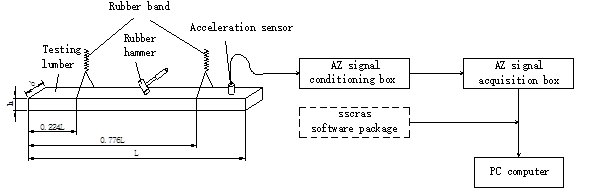Fig. 1. Test set-up for measuring the natural frequency of SPF lumber

The measurement set-up consisted of the following:

● One set of Nanjing Analyzer (Nanjing Analyzer Software Engineering Co., Ltd.; Nanjing, China) CRAS (Chinese Random Analysis System) vibration and dynamic signal acquisition and analysis system, including conditioning box, acquisition box, software SSCRAS (Signal System of Chinese Random Analysis System), and computer;

● One CA-YD-125 type piezoelectric accelerating speed sensor with 0.089 pC/ms-2 charge sensitivity and 1.5g of mass (Jiangsu Lianneng Electronic Technology Co., Ltd.; Yangzhou, China);

● One rubber hammer;

● One hammer equipped with force sensor (Langsi Measuring and Testing Technology Co., Ltd.; Qinhuangdao, China);

● One 30 kg electronic balance;

● One Guangzhuo type 701 outside micrometer (0~25 mm) (Guangzhuo Precision Instrument Co., Ltd.; Guanghou, China);

● One Kanghong steel tap (Kanghong Hardware Measuring Tool Co., Ltd.; Yucheng, China);

● One HK-30 wood moisture tester (Shanghai Jiashi Electronic Technology Co., Ltd.; Shanghai, China).

Test setup of modal test

The key for accurately deriving a MOE value from the test lumber was to determine the natural frequency of the first bending mode in a free beam condition. There was actually no real free beam, so a modal test (Ilic 2003; Zhang 1992) was first conducted to verify the free suspension of the test lumber with the frequency measurement and to identify the natural frequency of the first bending mode from the spectrum. The objective of the modal test was to apply an instantaneous aroused frequency method to obtain the MOE of the SPF dimensional lumber with high precision. The modal test set-up is shown in Figs. 2 and 3.

A Nanjing Analyzer CRAS was adopted as the hardware and MaCras (Mechanical and Structural model analysis software, V7.1; Nanjing Analyzer Software Engineering Co., Ltd.; Nanjing, China) as the software for the modal test. Parameter settings for the modal test were as follows: analysis frequency, 500 Hz; size of FFT (Fast Fourier Transformation) block, 1024 points; correction factor CH1, 32.8 mv/N and CH2, 8.9 mv/ ms-2 ; trigger mode, negative (triggering level 10% and trigger delay -20); voltage range, ±1250 mv (4 times programmable gain); and smoothing frequency, 500 Hz.

The test lumber was divided into 10 equal parts along the length. The test was performed by fixing the response point and moving the strike point.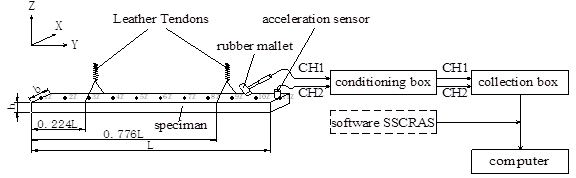Fig. 2. Modal test set-up for SPF lumber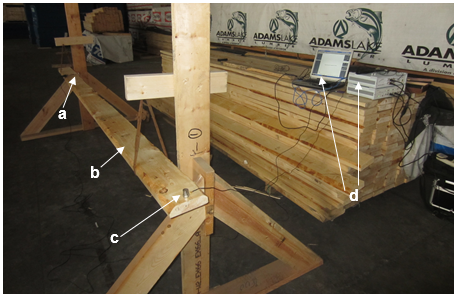Fig. 3. The modal test apparatus (Nanjing Cogenthome Manufactory Ltd.; Nanjing, China). (a) hammer strike (channel 1), (b) test SPF dimensional lumber, (c) acceleration sensor (channel 2), (d) CRAS vibration and dynamic signal acquisition and analysis system. The tested piece (b) does not even contact the vertical support.

Test setup of stress wave experiment

MOE values of the 300 test pieces of random SPF dimensional lumber were measured based on the beam transverse bending vibration theory. Other methods can also be used to verify the test results, such as the static method or the stress wave method based on a longitudinal vibration theory (Fu 2002). Considering the dimension of the test lumber was 3670 mm, static measurement for testing lumber in this mill was not available. Thus, a HM200 acoustic tool for log quality assessment (Hitman, Fibre-gen; Christchurch, New Zealand) was used to measure the lumber MOE (Wagner et al. 2000), as shown in Fig. 4. This tool measures the mean acoustic velocity along the lumber length. The lumber MOE can be estimated by multiplying lumber density by the square of the velocity (Ross et al. 1999).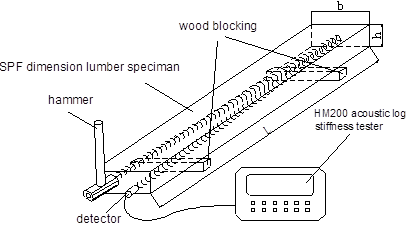Fig. 4. The stress wave test set-up for validating MOE in SPF dimensional lumber

Probability Distribution of the MOE of SPF Dimensional Lumber

Assume that the MOE of SPF dimensional lumber is a random variable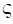, which is subjected to the following function of three-parameter Weibull distribution F(E) (Weibull 1951):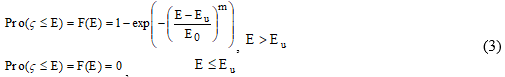where m, E0, and EU are distribution parameters in which m is the shape parameter, E0 is the scale parameter, and EU is the location parameter, indicating the minimum MOE among all the lumber samples.

Its probability density function f(E) can be expressed as follows: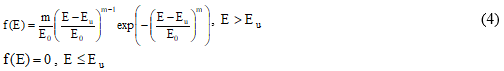With a gamma function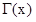, it is easy to derive the overall mean value μ and standard deviation σ: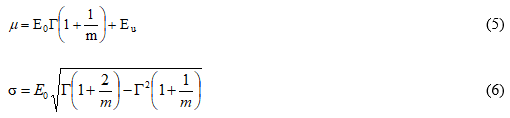Data Fitting for the SPF Lumber MOE

The measured MOE data of samples were first re-arranged in ascending order to form order statistics Ei, in which i=1, 2, 3……, 299, and 300. The corresponding probability value was i/(n + 1) (assuming that the order statistics Ei has the same value and k is its repetitions, the corresponding probability value will be (i + k)/(n + 1), in which n was the sample size, namely 300. For a given value of Ei, the following was used from formula (3):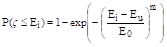(7)

Substitute the order statistics Ei and corresponding probability value i/(n + 1) into the above formula, and the following formula can be deduced: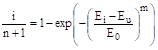(8)

that is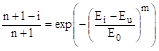(9)

Take the logarithm with a base of 10 on both sides, and one obtains: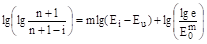(10)

First, take 0, 2000, 2500, 3500, 4000, 4500, and 5000 MPa for Eu according to the minimum value of actual MOE, 5526 MPa. Then calculate the value of point symmetry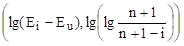in a Weibull coordinate system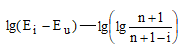. If a tested point symmetry falls in one line, the MOE data is considered to follow a Weibull distribution (Weibull 1951). Further, m is determined by the slope and E0 by the intercept between the straight line and vertical axis.

RESULTS AND DISCUSSION

Modal Test of SPF Lumber

The actual ratios of the first bending mode in the vibration and the bending frequency were very close to those theoretical values of a free beam test lumber. It was estimated that the interval for first bending frequency of the test lumber is about 11 to 15 Hz according to the modal test.

Figure 5 shows an example of the measured frequency and damping ratio for the first four bending modes. Using No. 26 SPF test lumber as an example, the distance between the node and both ends of the test lumber was 0.226L (the left) and 0.236L (the right), respectively. Such a result was quite close to the distance between the theoretical node and both ends (0.224L). The actual ratios of the second bending frequency, the third bending frequency, and the fourth bending frequency over the first bending frequency were 2.81, 5.55, and 9.15, respectively, while the theoretical ratios were 2.76, 5.40, and 8.93, respectively.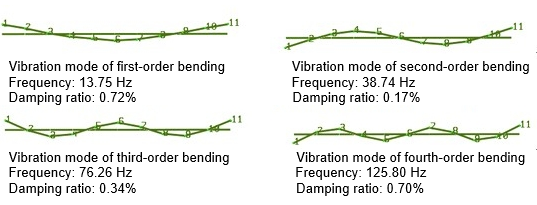Fig. 5. Measured frequency and damping ratio for the first four bending modes (e.g., No. 26 testing sample)

Result of First-order Natural Frequency of 300 Samples Measured by Transient Excitation Method

According to an analysis from the modal test, the MOE of the lumber was derived using the instantaneous aroused frequency method as follows:

1. Hang the test sample with a rubber band at a point 0.224l from each end to achieve a free beam condition. The modal test result showed that the suspension strength of the rubber band used in the test may be neglected.

2. Test the first bending frequency of the test sample in a single channel with an instantaneous hammer strike. Parameters for collection include: frequency, 50 Hz; FFT length, 2048 (frequency test precision can be up to 0.0652 Hz); voltage range, 1250 MV (four times programmable gain); amplification factor of conditioning instrument, 100; and smoothing frequency, 50 Hz to avoid frequency alias and ensure that there is no alias frequency (pseudo-frequency) of the third natural frequency in the spectrum.

3. Install an accelerometer at the left or right end of test sample and strike at the mid-span.

4. Use oscillography before formal data acquisition and strike the test sample continuously to check if the waveform is rational. If not, parameters should be reset. Acquire vibration signals and conduct a spectral analysis on the signals to read out the fundamental frequency in the linear spectrum (Fig. 6). The measurement should be repeated for each test sample. The mean value of frequency should be used to calculate lumber MOE.

According to the experimental scheme suggested above, the first bending frequency of the 300 SPF dimensional lumber specimens was tested. The result showed that overall samples had an average moisture content of 24%, of which the variable coefficient was 0.11 with an air-dry density of 493 kg/m3 of which the variable coefficient was 0.092. As an example, Fig. 6 shows the frequency spectrum of No. 26 SPF test lumber. It was easy to find the first bending frequency from the spectrum, namely 13.88 Hz fundamental frequency. Note that there were two small peaks near 2 Hz, which are the translation and torsion modes for this test lumber.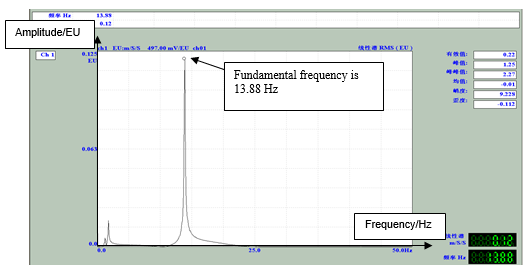Fig. 6. Measured spectrum of No. 26 SPF test lumber

Test Validation of Stress Wave Method

An HM200 acoustic tool was used to generate the data shown in Fig. 6. Figure 7 shows good correlation between MOE values measured by the frequency method and the stress wave (acoustic) method, providing an r of 0.96. The result demonstrates that the free beam vibration method is feasible for measuring lumber MOE. This method not only provided good repeatability and fast measurement, but also validated correctness of result measured by transient excitation frequency method with free suspension.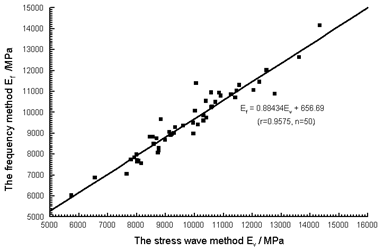Fig. 7. The MOE correlation between the frequency method and stress wave method

Evaluation of SPF Dimensional Lumber

When Eu=4500 MPa, a scatter plot of tested points in the Weibull coordinate system can be plotted as shown in Fig. 8.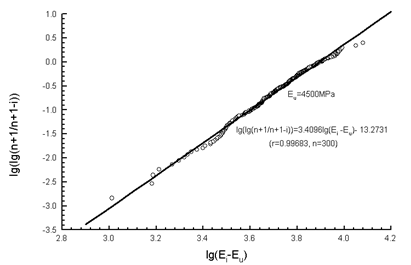Fig. 8. Fitting the measured SPF lumber MOE data with a Weibull distribution

When Eu=4500 MPa, the fit was quite good, providing a coefficient of determination of 0.997 (n=300). Therefore, the MOE of the SPF dimensional lumber can be accurately described with a Weibull distribution (Weibull 1951). Based on Fig. 8, the shape parameter m was about 3.4096 and scale parameter E0 was about 6118 MPa. Thus, the distribution function of the MOE of the SPF dimensional lumber can be expressed as follows：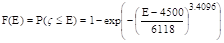(11)

The probability density function is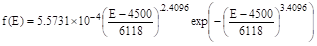(12)

Substitute m=3.4096, E0=6118 MPa, and Eu=4500 MPa into Eqs. 5 and 6 to obtain the following values. Based on the 300 lumber samples, the sample mean value was 10001 MPa with a standard deviation of 1822 MPa. It was estimated that the overall mean value μ and standard deviation σ are: μ=9997 MPa and σ=1781 MPa, with a coefficient of variation of 17.8%.

For any given value of location parameter Eu, Table 1 shows the possible Weibull parameters for the SPF dimensional lumber.

Table 1. Estimated Weibull Parameters Based on the Testing Data of the SPF Lumber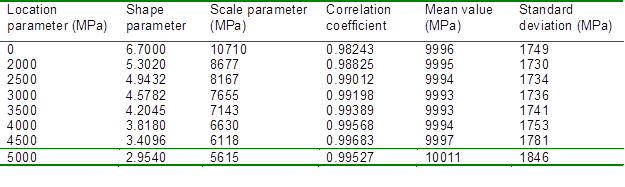In general, there are two cases for the testing of random variable distribution. One approach is to estimate the distribution type with a known parameter, while the other is to estimate the unknown parameters first and then test which distribution type fits best.. Among a number of testing methods, the K-S test (Kolmogorov-Smirnov goodness-of-fit test) is the most common (Wu and Ye 1990). Test hypothesis H0: the overall MOE of the SPF dimensional lumber is subject to the Weibull distribution. H1: the overall MOE of the SPF dimensional lumber is not subject to the Weibull distribution.

The K-S test with the test hypothesis H0 was the case of an unknown parameter, so the parameter should be estimated first. Table 2 shows possible estimated values, with a distribution function assumed to derive one of these parameters. Then the difference value between the known distribution function and the empirical distribution function was calculated in an order statistic. It was the maximum absolute value that was the value of test statistics Dn. Finally, the critical value of the test statistics Dn, α was obtained according to the significance level α and critical value table for the K-S test of Weibull distribution when checking unknown parameters of sample capacity. If DnDn, α, then Hwill be accepted; otherwise it will be rejected.

For a known distribution function, a series of possible Weibull parameters were assumed. Results for the K-S test are summarized in Table 2.

Assuming that the mean value of samples and standard deviation are the estimated values of overall mean and standard deviation, the K-S test was conducted for such a known distribution function. The calculation of Dn was the same as above, while the value of Dn, α was checked when parameters of normal distribution were unknown. The test results for a normal distribution are also listed in Table 2.

The critical value Dn, α of Dn can be obtained by referring sample number and significance level α to Table 2 (Gumbel 1958).

• For Weibull distribution: α=0.05, n=300, when parameters are unknown, Dn, α= D300, 0.05=0.888/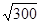=0.05127;
• For normal distribution: α=0.05, n=300, when parameters are unknown, Dn, α= D300, 0.05=0.886/=0.05115.

Table 2. K-S Inspection of Weibull Distribution and Normal Distribution with Unknown Parameters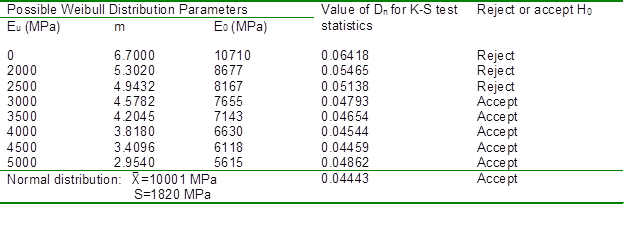From the results in Table 2, it can be seen that: (1) the MOE of the SPF dimensional lumber is not subject to the two-parameter Weibull distribution (Eu=0); (2) the MOE of the SPF dimensional lumber is likely to be fitted by a three-parameter Weibull distribution, in which location parameter Eu is larger than half of the minimum test value; and (3) the MOE of the SPF dimensional lumber is likely subject to a normal distribution.

Three-parameter Weibull distribution and normal distribution should be applied to estimate the probability for any given MOE values. The evaluation results are shown in Table 3.

Based on Table 3, the following can be observed:

1. The probability values calculated are stable and almost the same when 3500, 4000, and 4500 MPa are the given value of Eu. At this time, the given values of Eu are 63%, 72%, and 81% of 5526 MPa, the minimum MOE from the test, respectively. Therefore, it is recommended that the MOE with a value of 60% to 80% of the actual minimum MOE be used as of the location parameter Eu in the three-parameter Weibull distribution.

2. As shown in Fig. 9, the probabilities for the MOE of the SPF dimensional lumber calculated with a given value are almost equal in the three-parameter Weibull distribution and the normal distribution.

Table 3. Estimated Probability for Any Given MOE Values of SPF Dimensional Lumber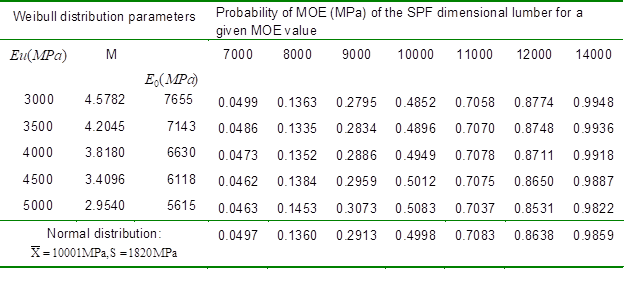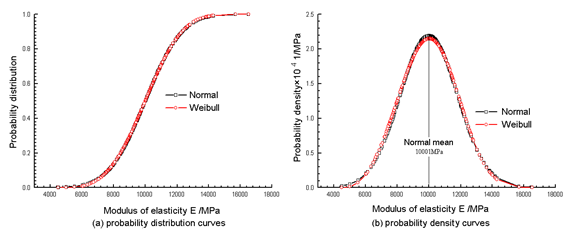Fig. 9. The distribution of the MOE value of the SPF dimensional lumber

From Fig. 9, it can be found that: (1) the probability density in Weibull is slightly asymmetric to its mean value (9997 MPa), which is different from the probability density curve in a normal distribution; and (2) the probability curve in the normal distribution is a little higher than that in the Weibull distribution when the elastic modulus is less than 7000 MPa, or, to the contrary when the elastic modulus is more than 7000 MPa.

Based on Canadian No. 2 dimensional lumber, the MOE value should be between 8000 and 9000 MPa as stated in GB 50005 (2003). Therefore, only the minimum 8000 MPa was considered when evaluating No. 2 lumber. Based on the three-parameter Weibull distribution, it was estimated that the probability for the MOE of the SPF dimensional lumber less than 8000 MPa (E= 4500 MPa) was about 13.8%. By comparison, the probability calculated based on the normal distribution was 13.6%. Thus, the difference seemed very small between those two distributions.

CONCLUSIONS

1. Based on this work, a reliable non-destructive testing (NDT) method was developed to measure the MOE of SPF dimensional lumber.
2. A modal test was first used to achieve a free suspension for the vibration test. The first natural frequency values from the bending mode of the SPF lumber was derived to calculate their MOE values.
3. The results were also validated by a stress wave method. The measured lumber MOE data was further fitted using a Weibull distribution and a normal distribution.
4. The results showed that the MOE of the SPF dimensional lumber followed either a three-parameter Weibull distribution or a normal distribution.
5. It was estimated that the probability of the MOE of this SPF dimensional lumber less than 8000 MPa (No. 2 SPF boundary value) is about 13.8% and 13.6%, respectively, using the above two distributions.

ACKNOWLEDGEMENTS

This study was funded by the Priority Academic Program Development of Jiangsu Higher Education Institutions (PAPD) and the Jiangsu Province Science and Technology Development Plan (BC2012416).

REFERENCES CITED

Brashaw, B. K., Bucur, V., Divos, F., Goncalves, R., and Lu J. X. (2009). “Nondestructive testing and evaluation of wood: a worldwide research update,” Forest Product Journal 59(3), 7-14.

Brown, L. S., DeVisser, D. A., Tuvey, R. S., and Rozek, A. K. (1997). “Mechanically graded lumber: The grading agency perspective,” Wood Design Focus 8(2), 3-7.

Cheng, K., and Timoshenko, S. (1965). Textbook of Mechanical Vibrational Science, Mechanical Industry Press, Beijing.

Fu, Z. F. (2002). Textbook of Modal Analysis of Vibration and Parameter Identification, Chinese Machine Press, Beijing.

GB 50005. (2003). “Code for design of timber structures,” The Ministry of Construction of the People’s Republic of China, China Construction Industry Press, Beijing.

GB/T 1933 (2009). “Method for determination of the density of wood,” China National Standardization Management Committee, China.

Green, D. W., Falk, R. H., and Lantz, S. F. (2001) “Effect of heart checks on flexural properties of reclaimed 6 by 8 Douglas-fir timbers,” Forest Products Journal 51(7-8), 82-88.

Gumbel, E. J. (1958). Textbook of Statistics of Extremes, Columbia University Press, New York.

Ilic, J. (2003). “Dynamic MOE of 55 species using small wood beams,” Holz als Roh-und Werkstoff61(3), 167-172.

Ridoutt, B. G., Wealleans, K. R., Booker, R. E., McConchie, D. L., and Ball, R. D. (1999). “Comparison of log segregation methods for structural lumber yield improvement,” Forest Products Journal 49(11-12), 63-66.

Ross, R. J., Willits, S. W., von Segen, W., Black, T., Brashaw, B. K., and Pellerin, R. F. (1999). “A stress wave based approach to NDE of logs for assessing potential veneer quality. Part 1. Small-diameter ponderosa pine,” Forest Products Journal 49(11-12), 60-62.

Wagner, F. G., Gorman, T. M., Layton, H. D., Rippy, R. C., and Bodenheimer, T. (2000). “Stress-wave analysis of Douglas-fir logs for veneer properties,” Forest Products Journal 50(4), 49-52.

Wang, X. P., Carter, P., Ross, R. J., and Brashaw, B. K. (2007). “Acoustic assessment of wood quality of raw forest materials: A path to increase profitability,” Forest Products Journal 57(5), 6-14.

Wang, Z. (2007). “Dynamic measure of elasticity model and damp ratio to HDF and OSB,” Journal of Nanjing Forestry University 31(3), 147-149.

Wang, Z., Li, L., and Gong, M. (2012). “Dynamic modulus of elasticity and damping ratio of wood-based composites using a cantilever beam vibration technique,” Construction & Building Materials28(1), 831-834.

Weibull, W. (1951). “A statistical distribution function of wide applicability,” Journal of Applied Mechanics-Transactions of the ASME 18(3), 293-297.

Wu, S. W., and Ye, J. (1990). “Analysis on critical values of K-S test methods of unknown parameters,” Tian Jin: Port Engineering Technology (1), 16-20.

Yin, Y. F., Lu J. X., Ni, C., Ren, H. Q. (2007). “Evaluation of bending, tensile and compressive strength of structural lumber with transverse vibration technique,” Journal of Building Materials10(5), 543-547.

Zhang, L. M. (1992). Textbook of Vibration Measurement and Dynamic Analysis, Aircraft Industry Press, Beijing.

Zheng, D. L., Li, Z. F., and Hua, H. X. (2002). “Status and trends of early vibration diagnosis technique of structures,” Journal of Vibration and Shock (2), 1-6.

Zhu, X. D., Wang, F. H., Cao, J., and Sun, J. P. (2010). “Nondestructive test system for wood vibration based on virtual instrument,” Journal of Northwest Forestry University 25(5), 182-186.

Article submitted: February 21, 2014; Peer review completed: April 4, 2014; Revised version received and accepted: May 5, 2014; Published: May 9, 2014.